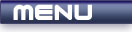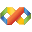Home Latest News Archive Tutorials C++ 2 3 4 Win32 Java OpenGL GLUT OpenGL ES 2 3 4 DirectX VB.NET HTML Photoshop 3dsmax Forums Projects Modelling Links About Us Contact Search Recent Tutorials C / C++ Win32 OpenGL OpenGL ES GLUT## Passing Parameters

Tutorials > C++ > Passing Parameters

### What happens when we pass parameters?

When you pass a parameter as shown in previous tutorials, what happens is that a copy of the variable is created and the value is used. This is known as passing parameters by value. This means that the value of your variable before calling the function will be the same even if this value is modified within the function.

If you want to retain the modified value, you need to pass variables by reference. This can be done either using pointers or references. Both cases will be covered in this tutorial.

Contents of main.cpp :

```#include <iostream>
#include <stdlib.h>

using namespace std;
```

Our first function shows a paramater being passed by value. The value of x is increased by 5 and then the value is displayed.

```void add5ByValue(int x)
{
x += 5;

cout << "x in function : "
<< x << endl;
}
```

Our next function shows a parameter being passed by reference using pointers. There are only two differences. The one difference is that the function requires a memory address to be passed and the other difference is that the variable must always be dereferenced when operating on the value that the memory address points to.

```void add5ByRefUsingPointers(int *x)
{
*x += 5;

cout << "x in function : "
<< *x << endl;
}
```

Our next function shows a parameter being passed by reference using references. There is only one difference. The parameter needs to be declared as a reference instead of a normal variable. The variable can then be used as per normal.

```void add5ByRefUsingReferences(int &x)
{
x += 5;

cout << "x in function : "
<< x << endl;
}
```

We can now see how all these functions act in the code segments below. x is initialized with a value of 0.

```int main()
{
int x = 0;
```

First we pass our variable by value. The message is printed inside the function which shows that x is equal to 5. The value of x is then displayed after the function call. We find that the value is still 0. This is because the variable was copied and the function was not actually working on the original variable. This is useful if we want to retain our original value.

```	add5ByValue(x);

cout << "x out of function : "
<< x << endl;

cout << endl;
```

We will now pass x by reference using pointers. Because the function requires a memory address, we need to use the & operator. When we print out the value of x after the function call, we notice that the modified value of 5 is retained.

```	add5ByRefUsingPointers(&x);

cout << "x out of function : "
<< x << endl;

cout << endl;
```

Passing parameters by reference using references gives the same result. The function requires a normal variable to be passed as the parameter is declared as being a reference. We can therefore pass the variable in the normal manner. We find that the modified value of 10 is retained.

```	add5ByRefUsingReferences(x);

cout << "x out of function : "
<< x << endl;

system("pause");

return 0;
}```

You should now understand how to pass parameters by value and by reference. You should also be able to pass parameters by reference using both pointers and references. Using references offers the advantage of not having to pass a memory address and not having to have constant dereferences. Hopefully you now see why references are so useful.

Please let me know of any comments you may have : Contact Me

 Source Files :< Tutorial 22 - References Tutorial 24 - Macros >